# NCERT Exemplar Solutions for Class 7 Maths Chapter 10 Algebraic Expressions

NCERT Exemplar Solutions for Class 7 Maths Chapter 10 Algebraic Expressions are available here. While solving the exercise questions from the NCERT Exemplar book, students often face difficulty and eventually pile up their doubts. Our expert tutors formulate these exercises to assist you with your exam preparation to attain good marks in Maths. Students who wish to score good marks in the subject practise NCERT Exemplar Solutions for Class 7 Maths.

Chapter 10 Algebraic Expressions explains about Mathematical expression that consists of variables, numbers, and operations. The value of this expression can change. Topics covered in this Chapter are listed below:

• Terms of An Expression
• Like and Unlike Terms
• Monomials, Binomials, Trinomials, and Polynomials
• Addition and Subtraction of Algebraic Expressions
• Finding The Value of An Expression

## Download the PDF of NCERT Exemplar Solutions for Class 7 Maths Chapter 10 Algebraic Expressions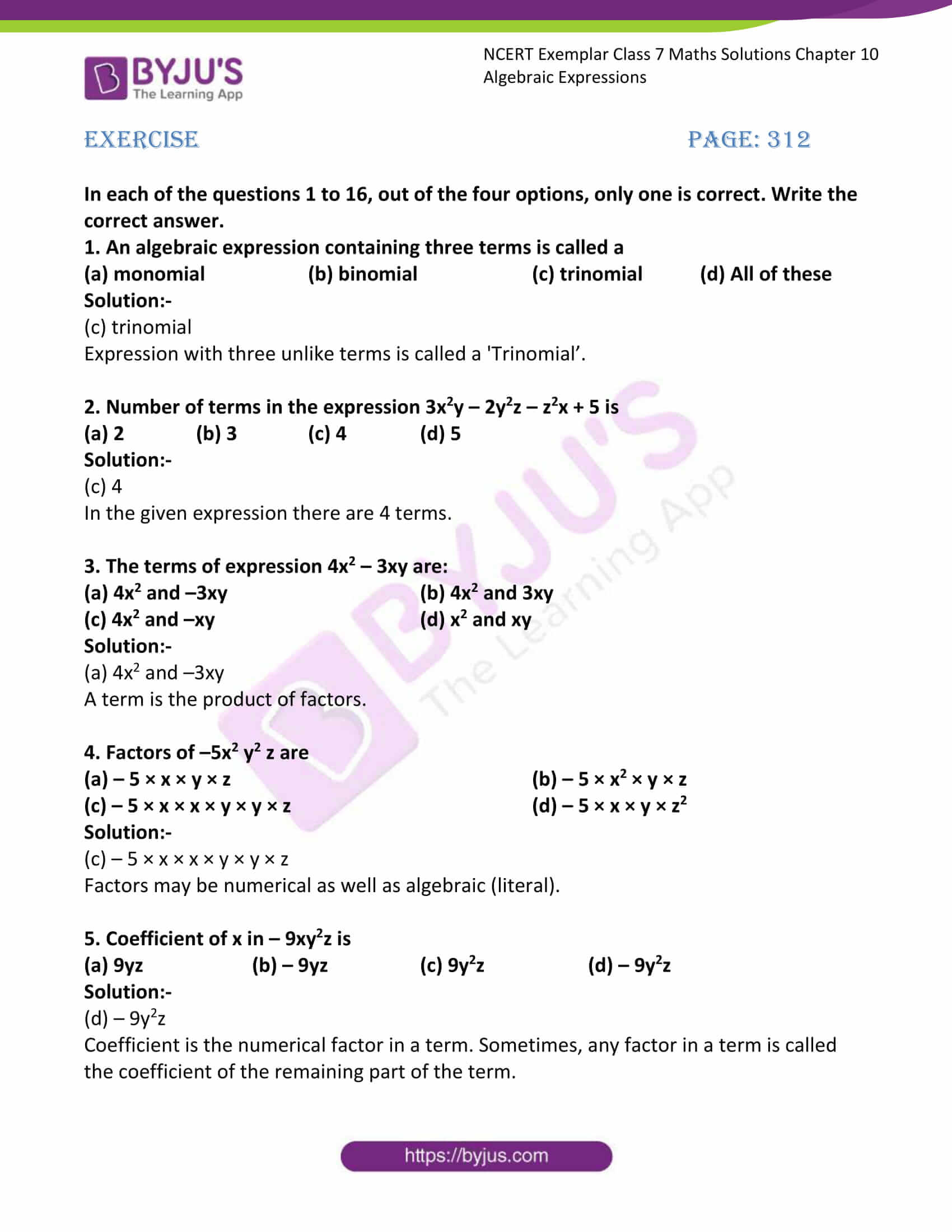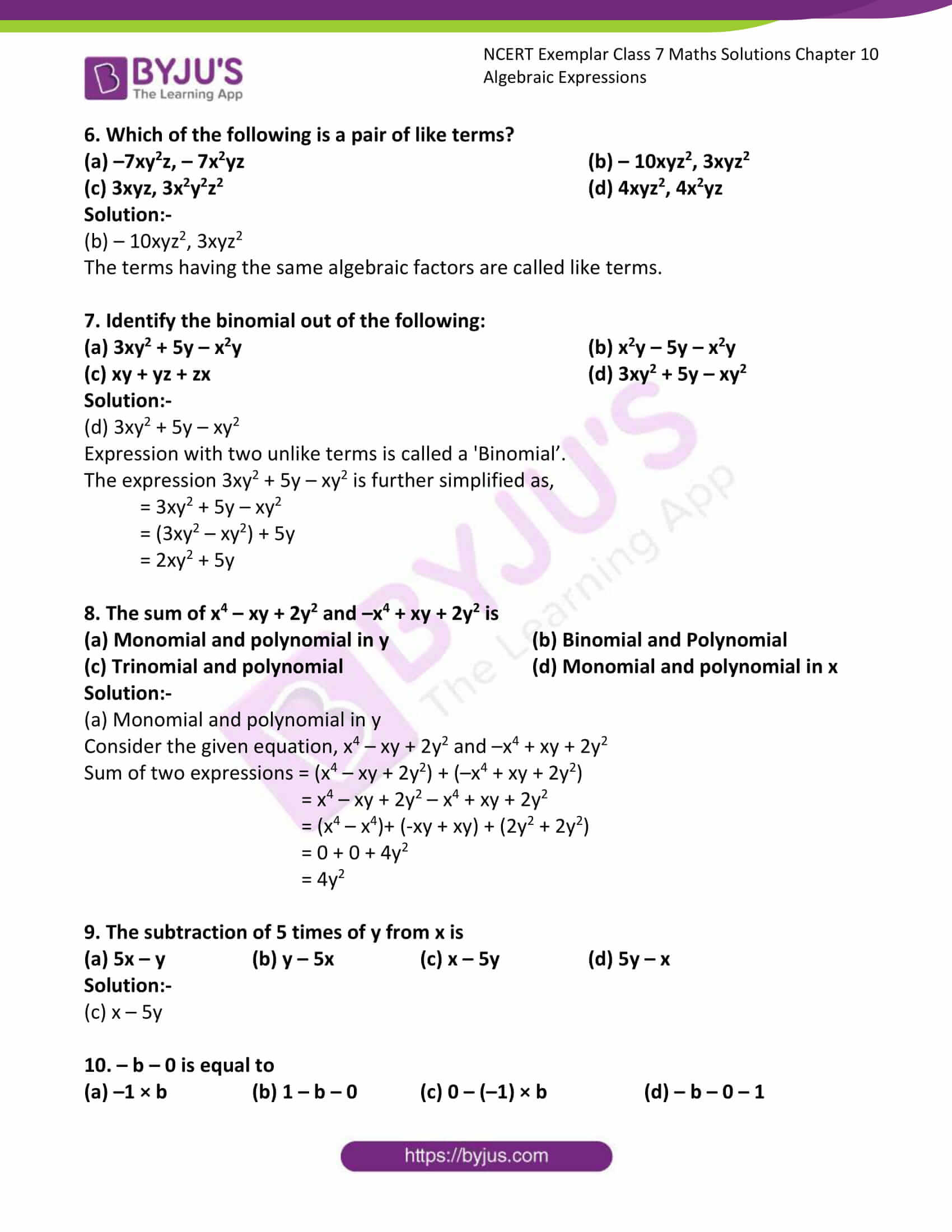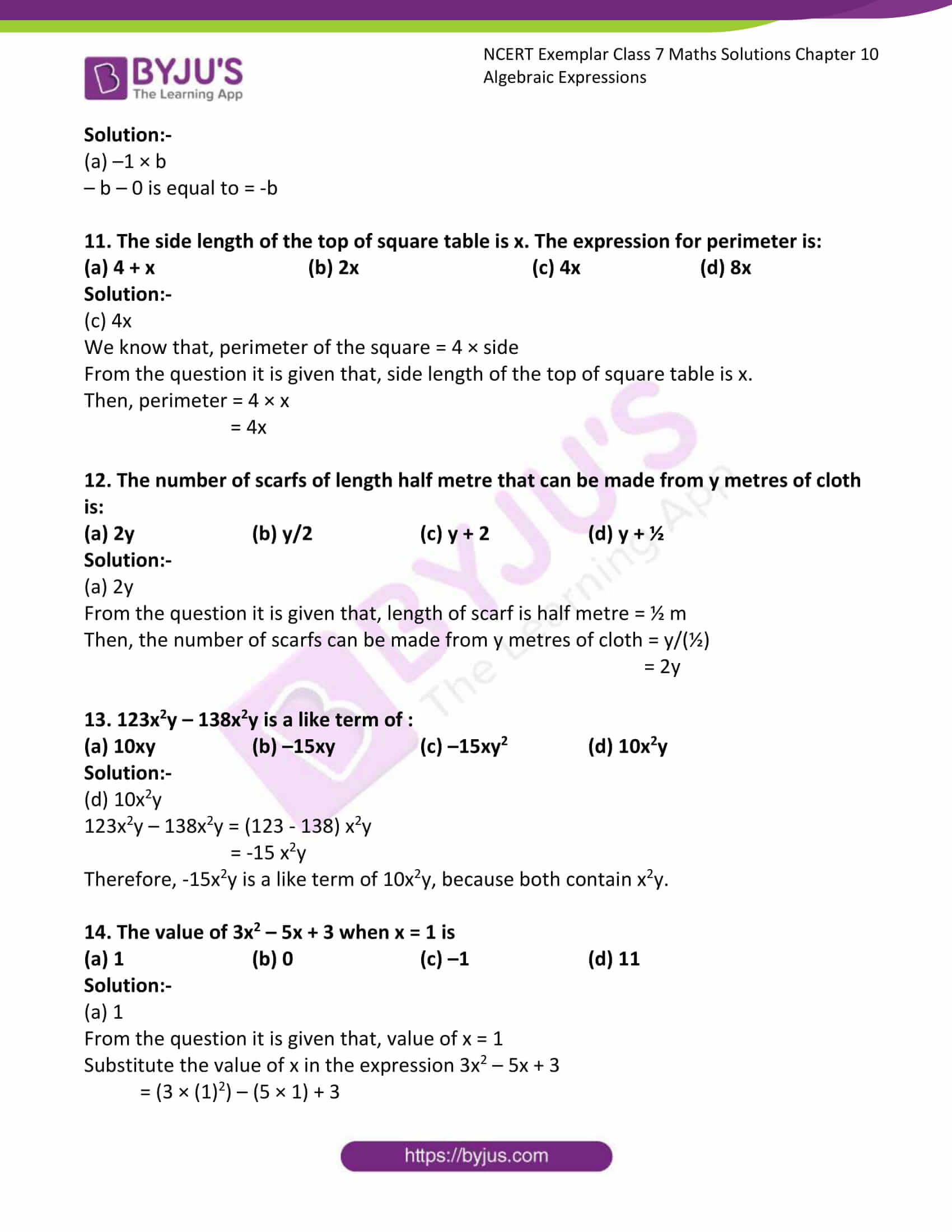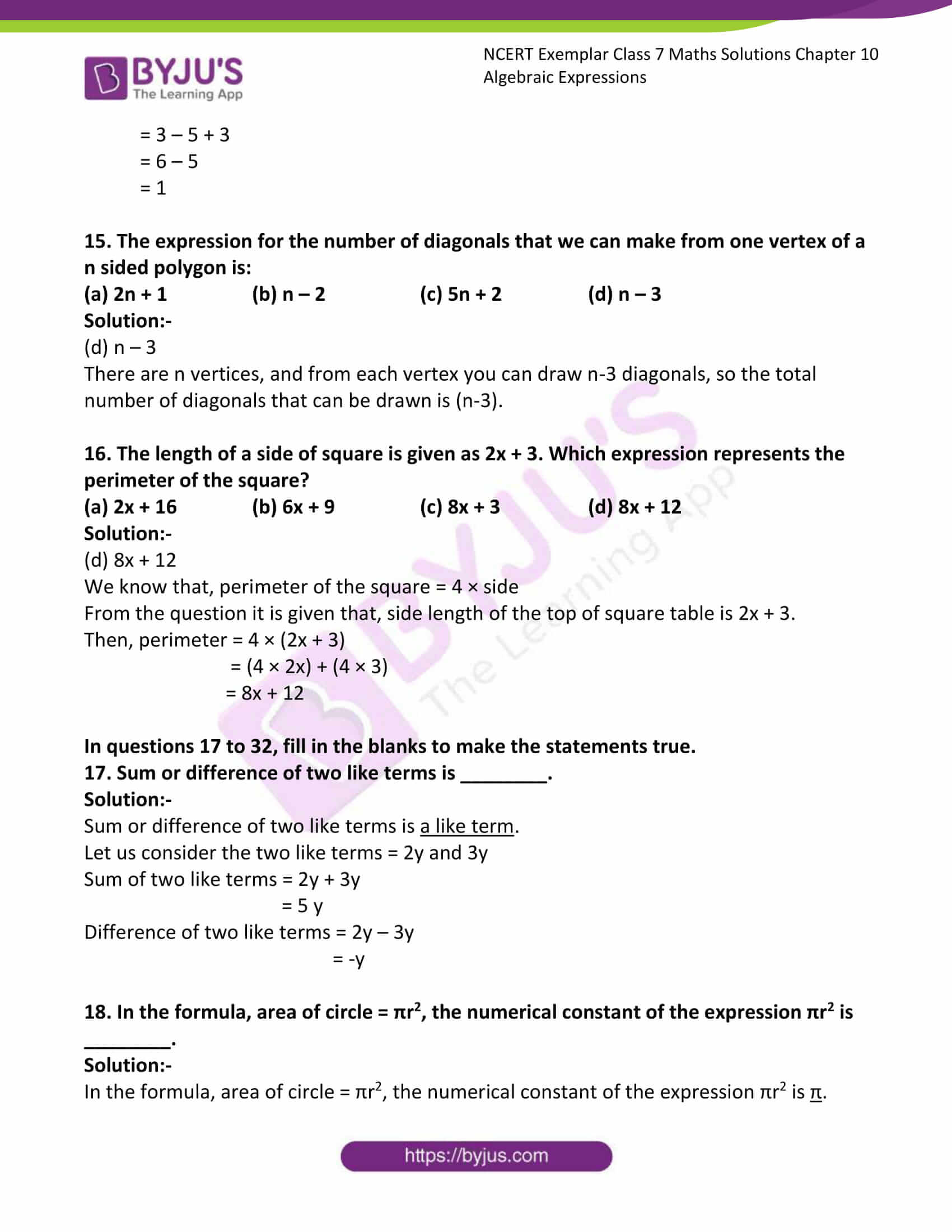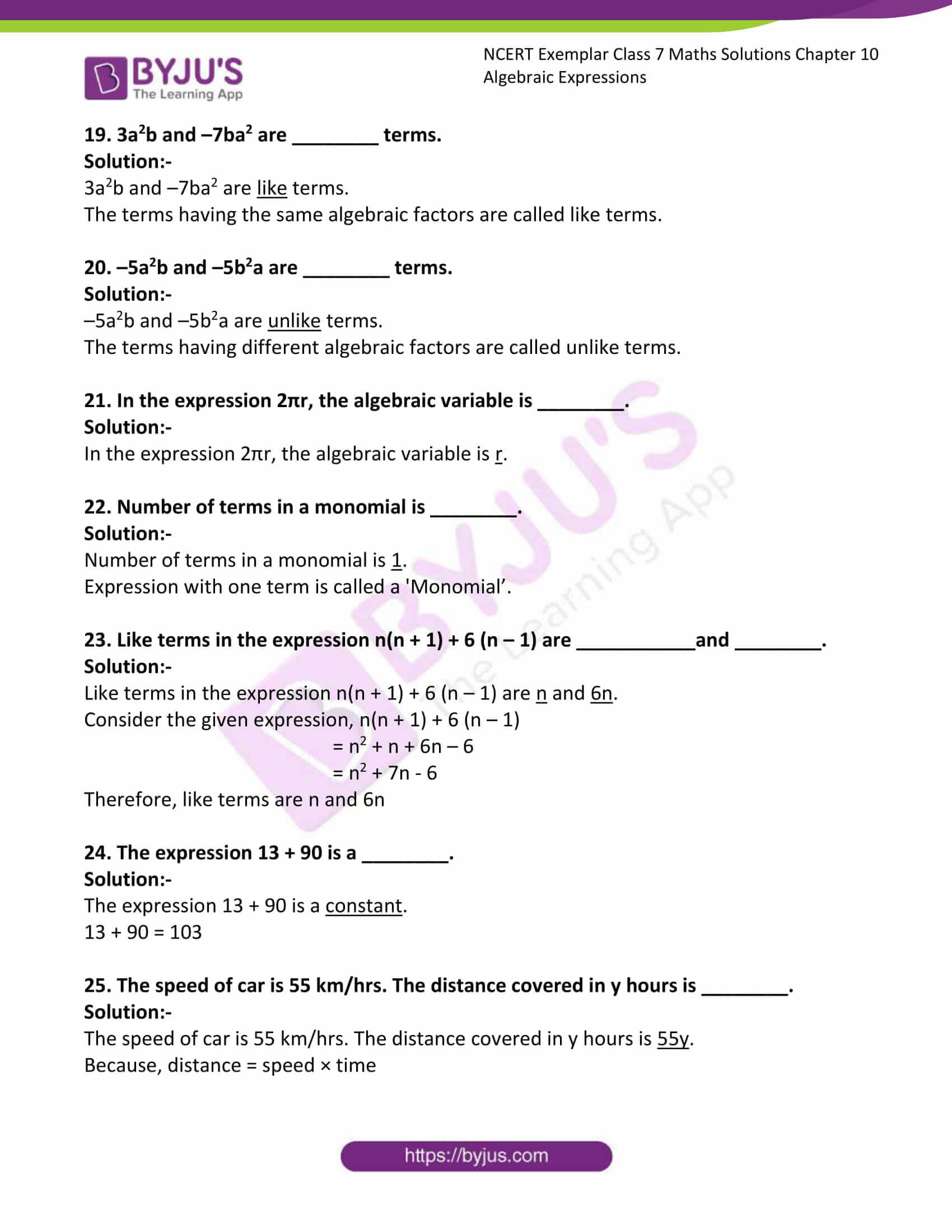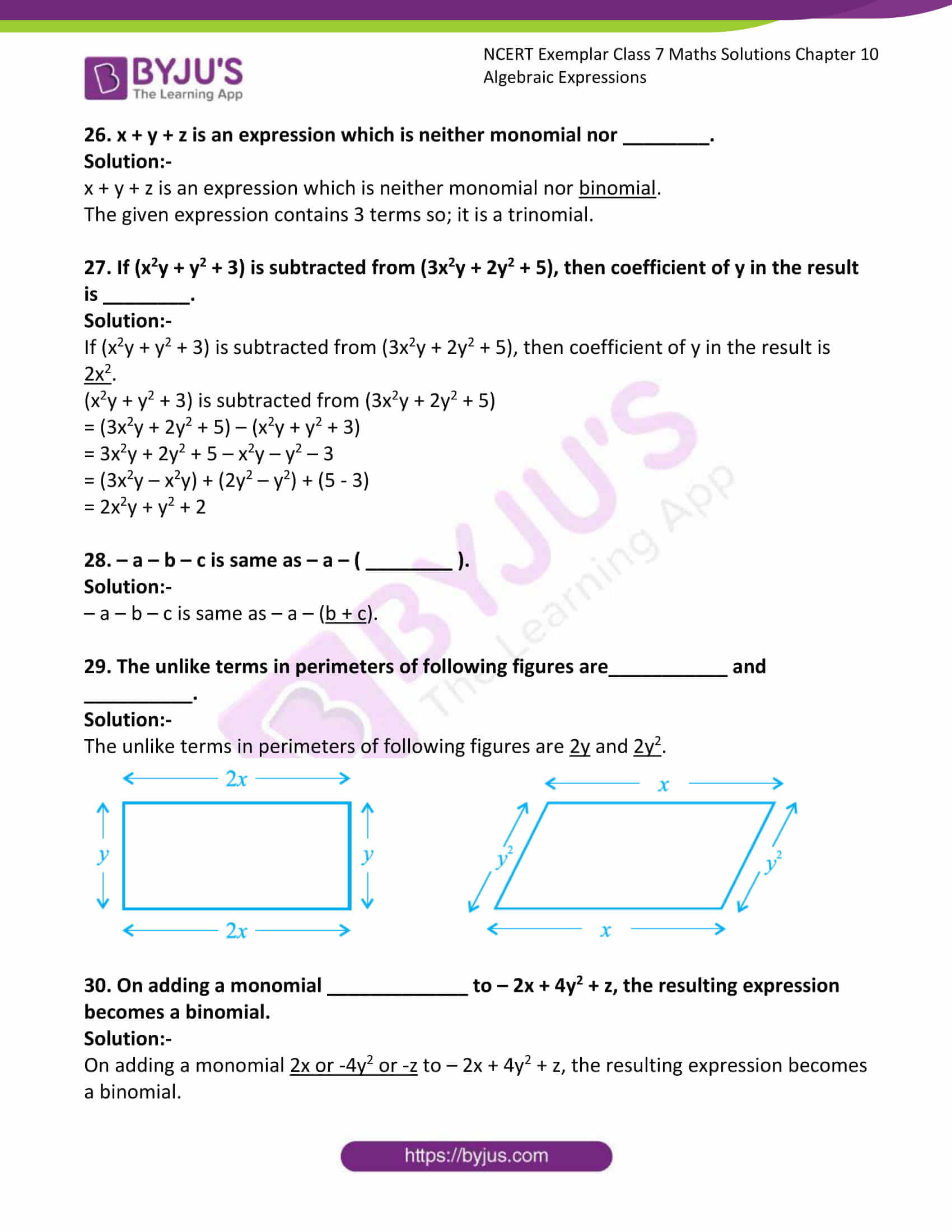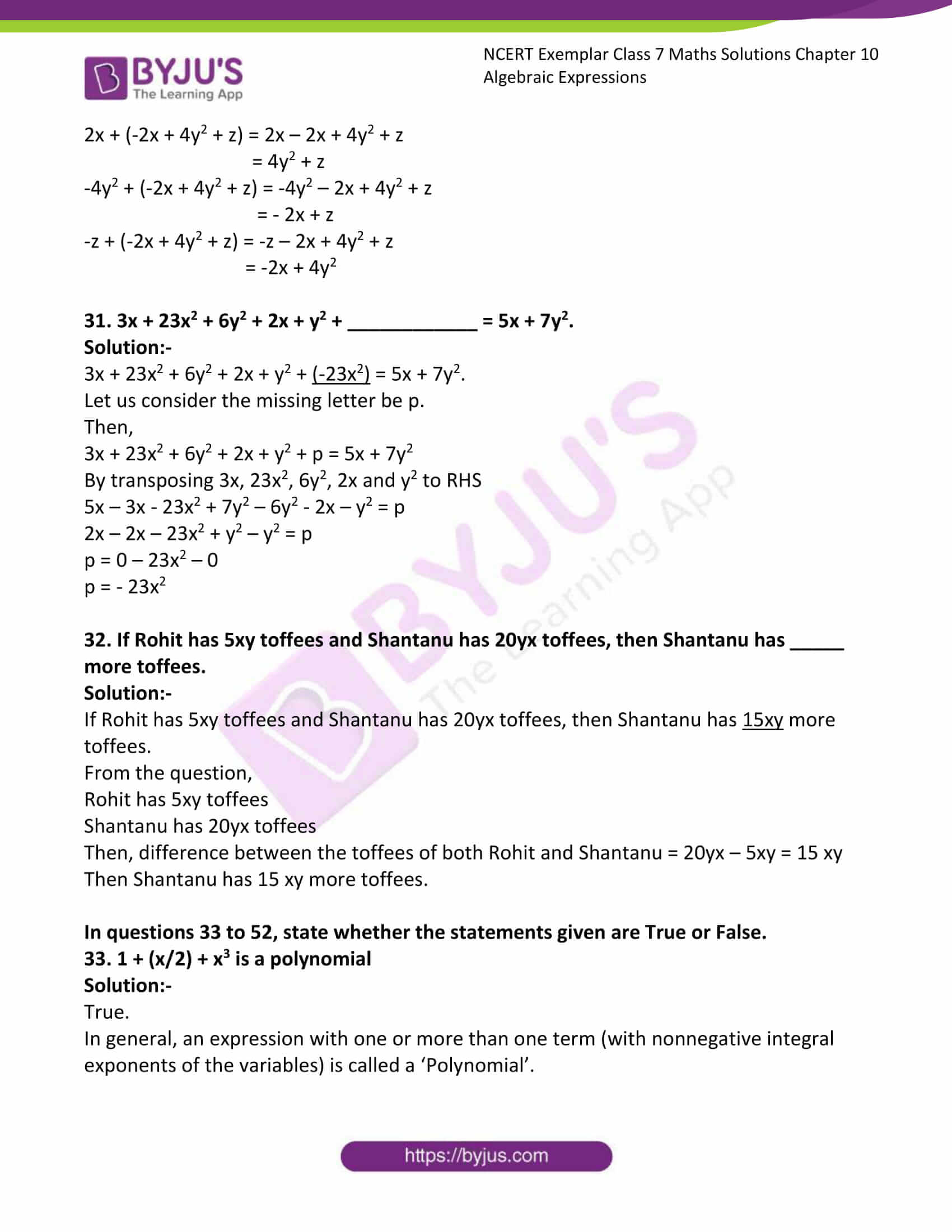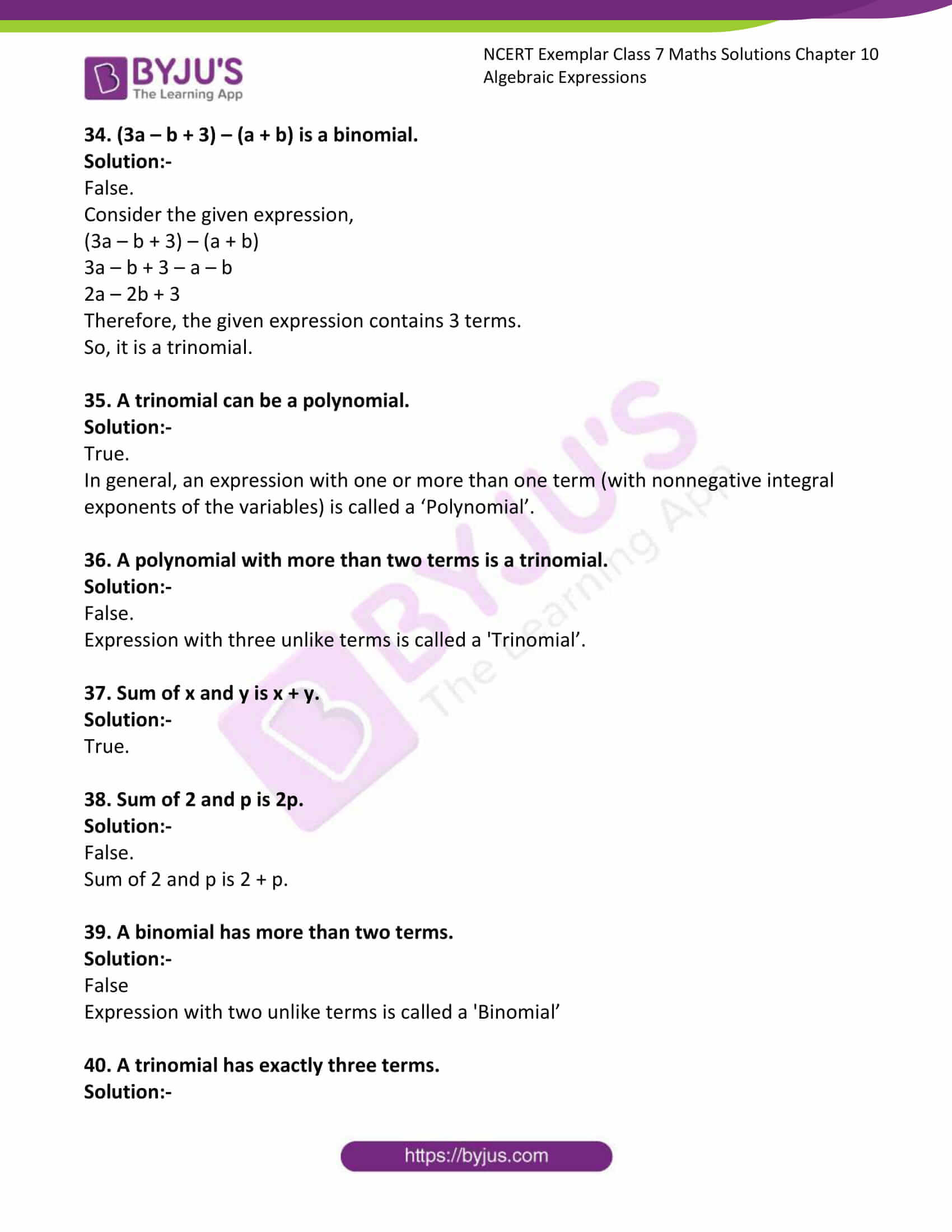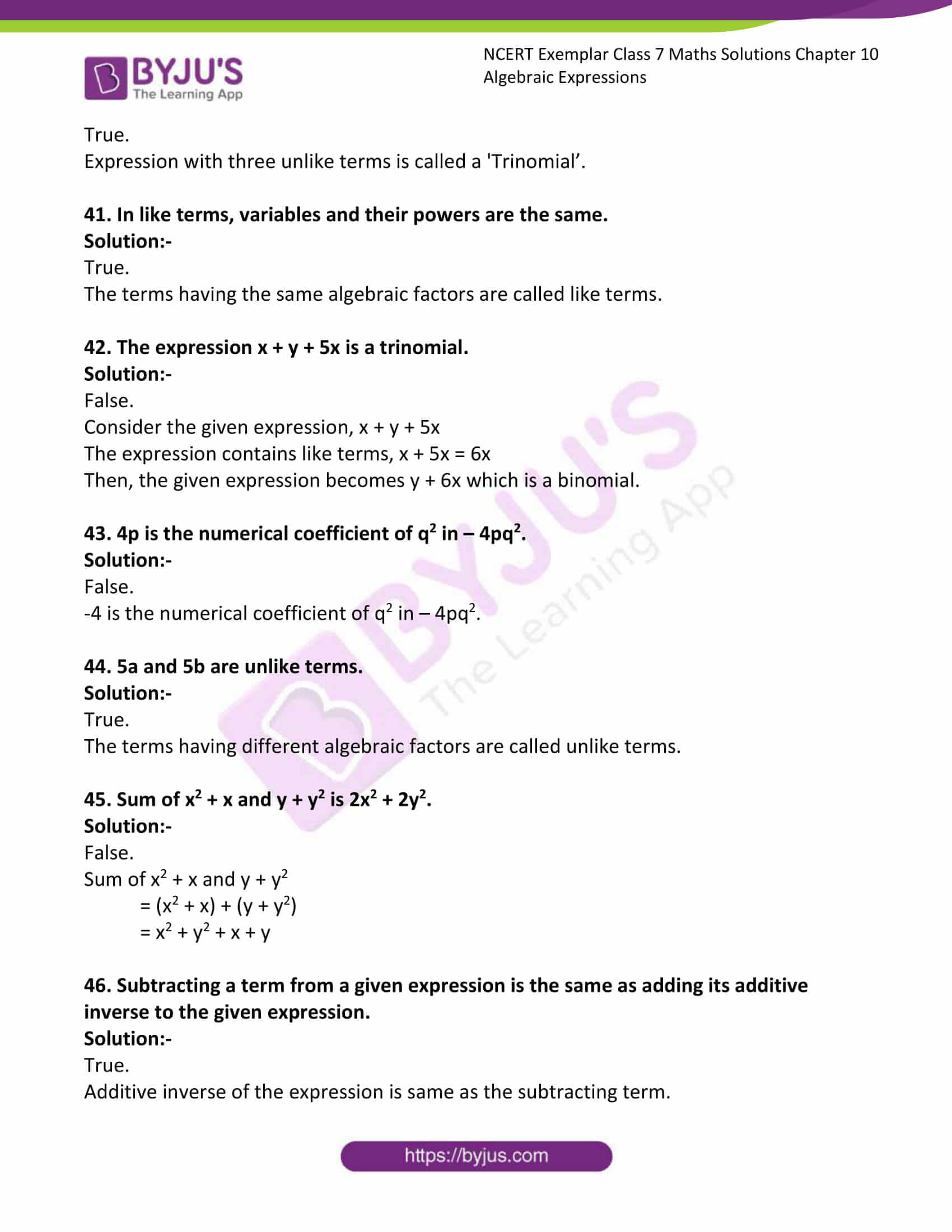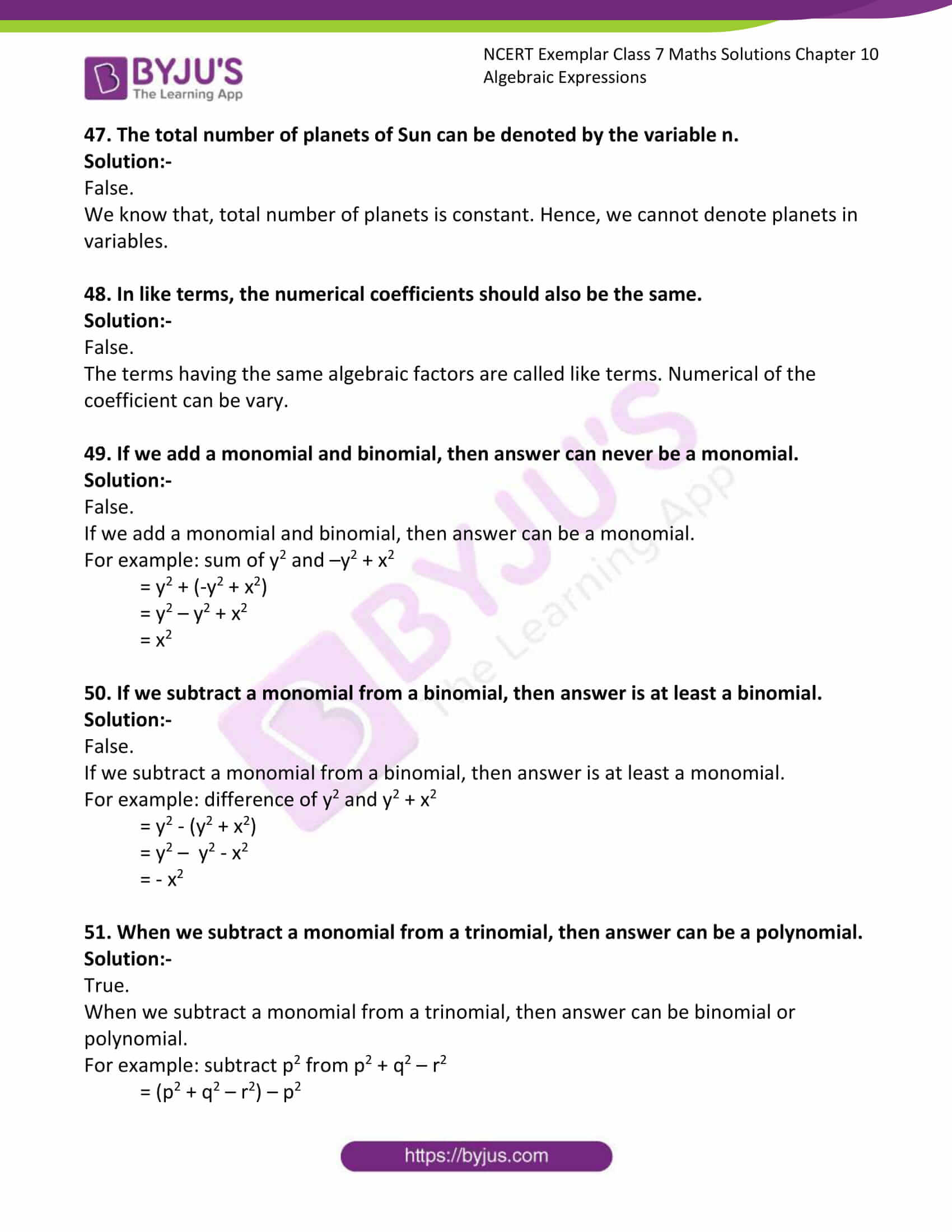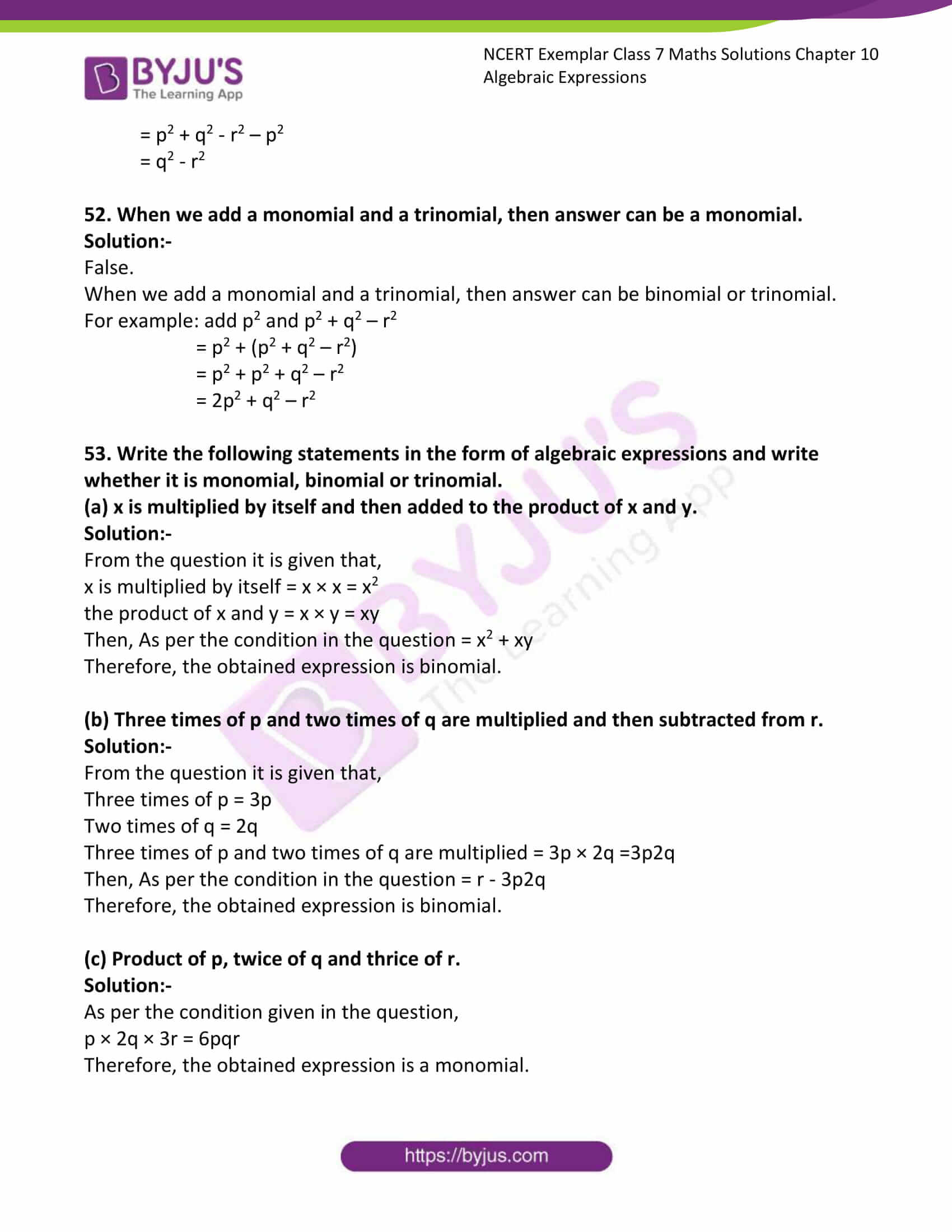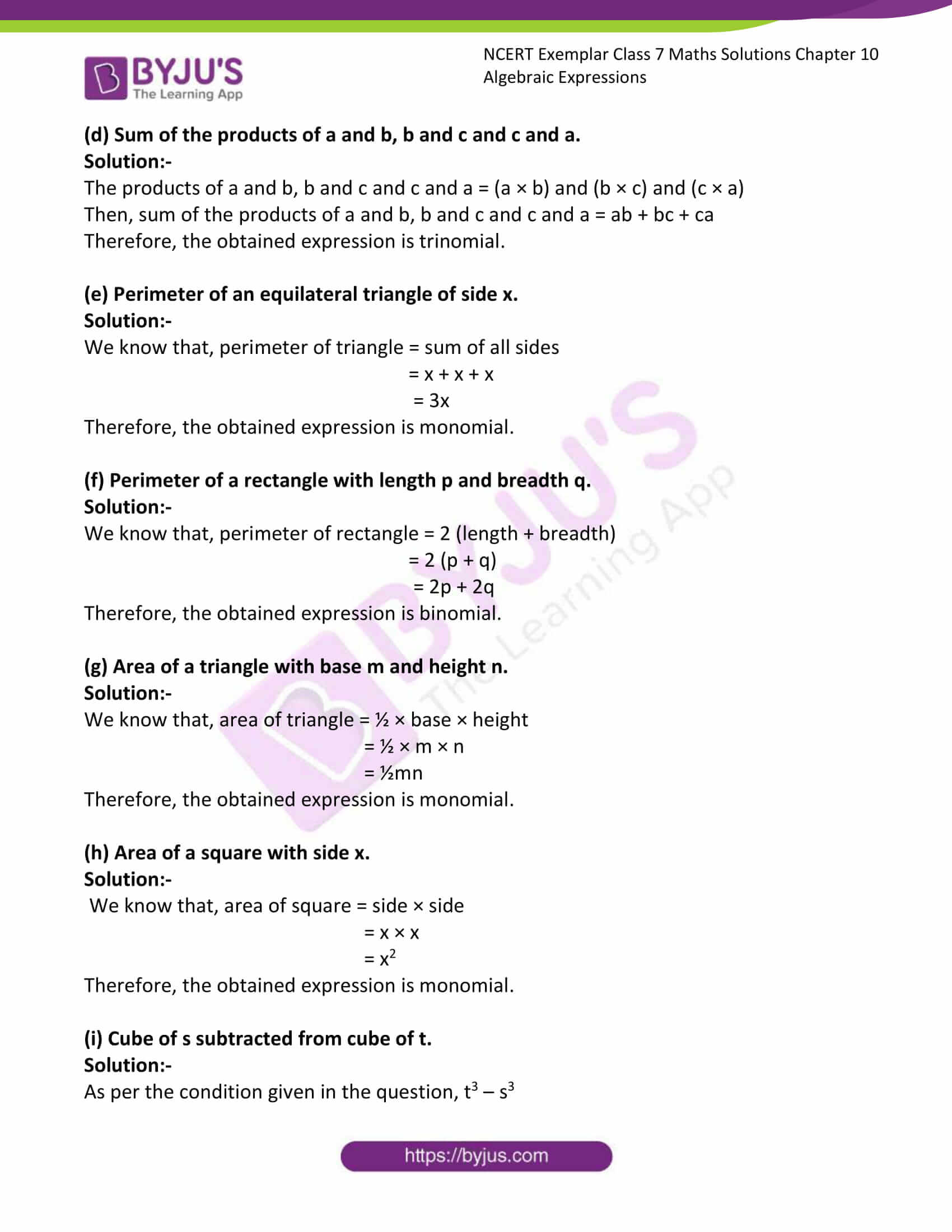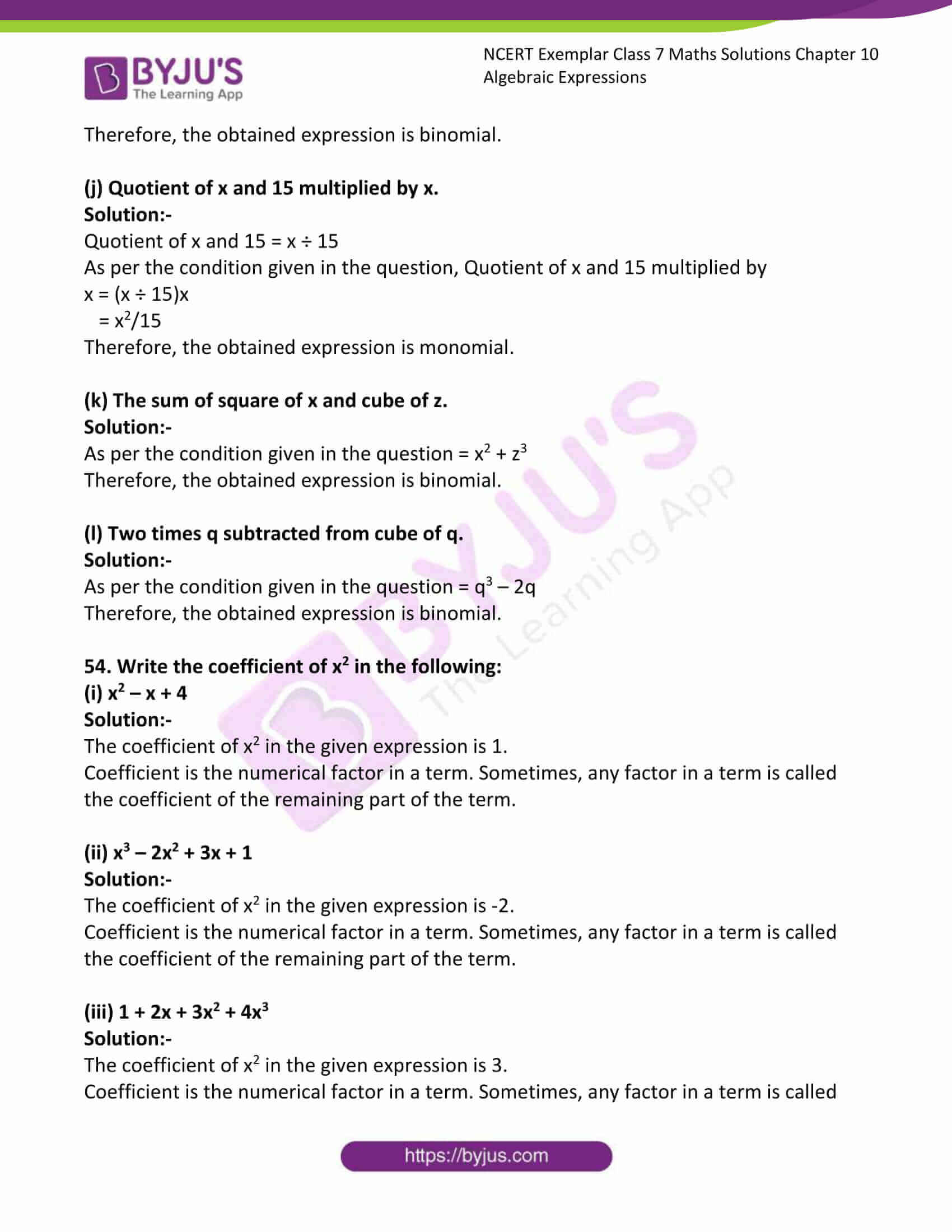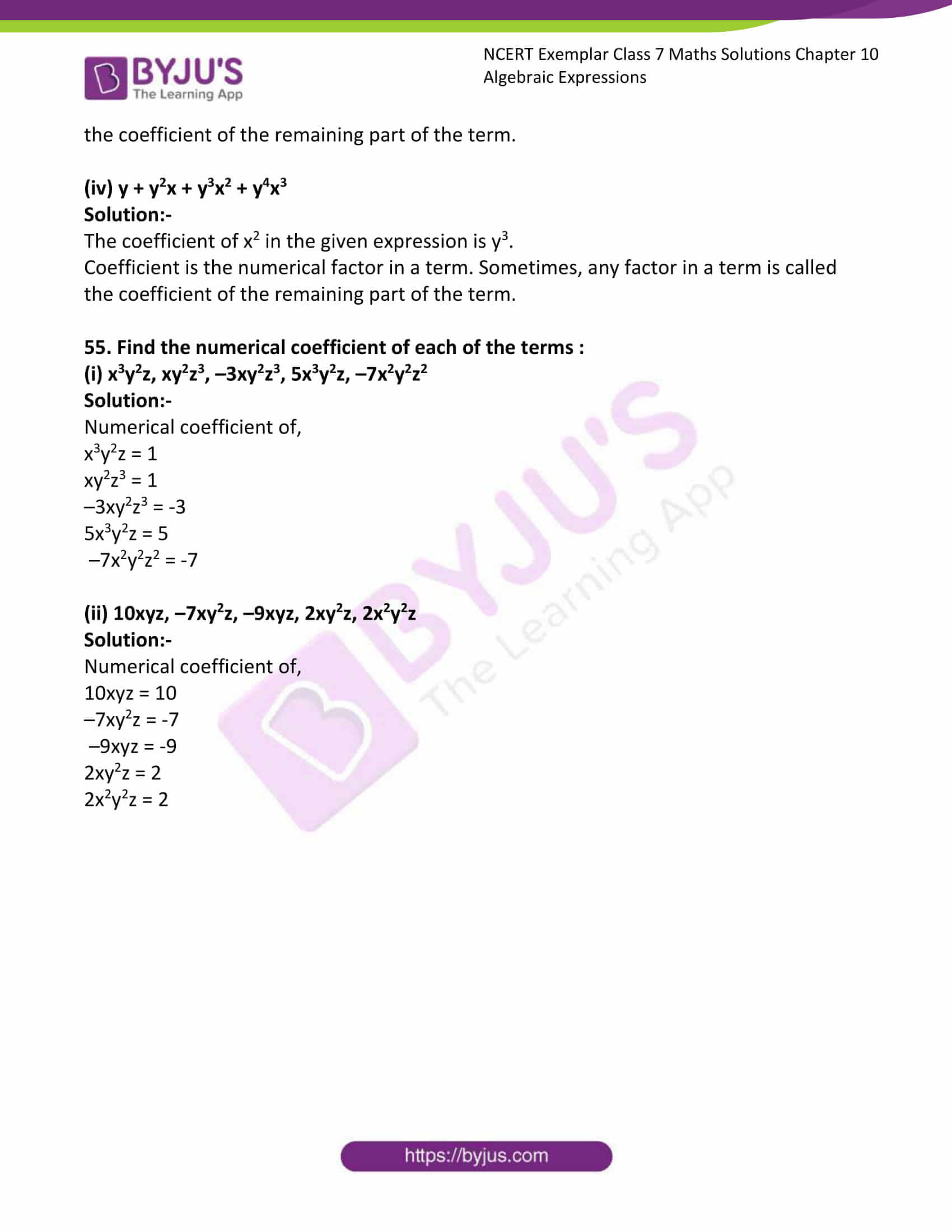### Access Answers to Maths NCERT Exemplar Solutions for Class 7 Chapter 10 Algebraic Expressions

Exercise Page: 312

In each of the questions 1 to 16, out of the four options, only one is correct. Write the correct answer.

1. An algebraic expression containing three terms is called a

(a) monomial (b) binomial (c) trinomial (d) All of these

Solution:-

(c) trinomial

Expression with three unlike terms is called a ‘Trinomial’.

2. Number of terms in the expression 3x2y – 2y2z – z2x + 5 is

(a) 2 (b) 3 (c) 4 (d) 5

Solution:-

(c) 4

In the given expression there are 4 terms.

3. The terms of expression 4x2 – 3xy are:

(a) 4x2 and –3xy (b) 4x2 and 3xy

(c) 4x2 and –xy (d) x2 and xy

Solution:-

(a) 4x2 and –3xy

A term is the product of factors.

4. Factors of –5x2 y2 z are

(a) – 5 × x × y × z (b) – 5 × x2 × y × z

(c) – 5 × x × x × y × y × z (d) – 5 × x × y × z2

Solution:-

(c) – 5 × x × x × y × y × z

Factors may be numerical as well as algebraic (literal).

5. Coefficient of x in – 9xy2z is

(a) 9yz (b) – 9yz (c) 9y2z (d) – 9y2z

Solution:-

(d) – 9y2z

Coefficient is the numerical factor in a term. Sometimes, any factor in a term is called the coefficient of the remaining part of the term.

6. Which of the following is a pair of like terms?

(a) –7xy2z, – 7x2yz (b) – 10xyz2, 3xyz2

(c) 3xyz, 3x2y2z2 (d) 4xyz2, 4x2yz

Solution:-

(b) – 10xyz2, 3xyz2

The terms having the same algebraic factors are called like terms.

7. Identify the binomial out of the following:

(a) 3xy2 + 5y – x2y (b) x2y – 5y – x2y

(c) xy + yz + zx (d) 3xy2 + 5y – xy2

Solution:-

(d) 3xy2 + 5y – xy2

Expression with two unlike terms is called a ‘Binomial’.

The expression 3xy2 + 5y – xy2 is further simplified as,

= 3xy2 + 5y – xy2

= (3xy2 – xy2) + 5y

= 2xy2 + 5y

8. The sum of x4 – xy + 2y2 and –x4 + xy + 2y2 is

(a) Monomial and polynomial in y (b) Binomial and Polynomial

(c) Trinomial and polynomial (d) Monomial and polynomial in x

Solution:-

(a) Monomial and polynomial in y

Consider the given equation, x4 – xy + 2y2 and –x4 + xy + 2y2

Sum of two expressions = (x4 – xy + 2y2) + (–x4 + xy + 2y2)

= x4 – xy + 2y2 – x4 + xy + 2y2

= (x4 – x4)+ (-xy + xy) + (2y2 + 2y2)

= 0 + 0 + 4y2

= 4y2

9. The subtraction of 5 times of y from x is

(a) 5x – y (b) y – 5x (c) x – 5y (d) 5y – x

Solution:-

(c) x – 5y

10. – b – 0 is equal to

(a) –1 × b (b) 1 – b – 0 (c) 0 – (–1) × b (d) – b – 0 – 1

Solution:-

(a) –1 × b

– b – 0 is equal to = -b

11. The side length of the top of square table is x. The expression for perimeter is:

(a) 4 + x (b) 2x (c) 4x (d) 8x

Solution:-

(c) 4x

We know that, perimeter of the square = 4 × side

From the question it is given that, side length of the top of square table is x.

Then, perimeter = 4 × x

= 4x

12. The number of scarfs of length half metre that can be made from y metres of cloth is:

(a) 2y (b) y/2 (c) y + 2 (d) y + ½

Solution:-

(a) 2y

From the question it is given that, length of scarf is half metre = ½ m

Then, the number of scarfs can be made from y metres of cloth = y/(½)

= 2y

13. 123x2y – 138x2y is a like term of :

(a) 10xy (b) –15xy (c) –15xy2 (d) 10x2y

Solution:-

(d) 10x2y

123x2y – 138x2y = (123 – 138) x2y

= -15 x2y

Therefore, -15x2y is a like term of 10x2y, because both contain x2y.

14. The value of 3x2 – 5x + 3 when x = 1 is

(a) 1 (b) 0 (c) –1 (d) 11

Solution:-

(a) 1

From the question it is given that, value of x = 1

Substitute the value of x in the expression 3x2 – 5x + 3

= (3 × (1)2) – (5 × 1) + 3

= 3 – 5 + 3

= 6 – 5

= 1

15. The expression for the number of diagonals that we can make from one vertex of a n sided polygon is:

(a) 2n + 1 (b) n – 2 (c) 5n + 2 (d) n – 3

Solution:-

(d) n – 3

There are n vertices, and from each vertex you can draw n-3 diagonals, so the total number of diagonals that can be drawn is (n-3).

16. The length of a side of square is given as 2x + 3. Which expression represents the perimeter of the square?

(a) 2x + 16 (b) 6x + 9 (c) 8x + 3 (d) 8x + 12

Solution:-

(d) 8x + 12

We know that, perimeter of the square = 4 × side

From the question it is given that, side length of the top of square table is 2x + 3.

Then, perimeter = 4 × (2x + 3)

= (4 × 2x) + (4 × 3)

= 8x + 12

In questions 17 to 32, fill in the blanks to make the statements true.

17. Sum or difference of two like terms is ________.

Solution:-

Sum or difference of two like terms is a like term.

Let us consider the two like terms = 2y and 3y

Sum of two like terms = 2y + 3y

= 5 y

Difference of two like terms = 2y – 3y

= -y

18. In the formula, area of circle = πr2, the numerical constant of the expression πr2 is ________.

Solution:-

In the formula, area of circle = πr2, the numerical constant of the expression πr2 is π.

19. 3a2b and –7ba2 are ________ terms.

Solution:-

3a2b and –7ba2 are like terms.

The terms having the same algebraic factors are called like terms.

20. –5a2b and –5b2a are ________ terms.

Solution:-

–5a2b and –5b2a are unlike terms.

The terms having different algebraic factors are called unlike terms.

21. In the expression 2πr, the algebraic variable is ________.

Solution:-

In the expression 2πr, the algebraic variable is r.

22. Number of terms in a monomial is ________.

Solution:-

Number of terms in a monomial is 1.

Expression with one term is called a ‘Monomial’.

23. Like terms in the expression n(n + 1) + 6 (n – 1) are ___________and ________.

Solution:-

Like terms in the expression n(n + 1) + 6 (n – 1) are n and 6n.

Consider the given expression, n(n + 1) + 6 (n – 1)

= n2 + n + 6n – 6

= n2 + 7n – 6

Therefore, like terms are n and 6n

24. The expression 13 + 90 is a ________.

Solution:-

The expression 13 + 90 is a constant.

13 + 90 = 103

25. The speed of car is 55 km/hrs. The distance covered in y hours is ________.

Solution:-

The speed of car is 55 km/hrs. The distance covered in y hours is 55y.

Because, distance = speed × time

26. x + y + z is an expression which is neither monomial nor ________.

Solution:-

x + y + z is an expression which is neither monomial nor binomial.

The given expression contains 3 terms so; it is a trinomial.

27. If (x2y + y2 + 3) is subtracted from (3x2y + 2y2 + 5), then coefficient of y in the result is ________.

Solution:-

If (x2y + y2 + 3) is subtracted from (3x2y + 2y2 + 5), then coefficient of y in the result is 2x2.

(x2y + y2 + 3) is subtracted from (3x2y + 2y2 + 5)

= (3x2y + 2y2 + 5) – (x2y + y2 + 3)

= 3x2y + 2y2 + 5 – x2y – y2 – 3

= (3x2y – x2y) + (2y2 – y2) + (5 – 3)

= 2x2y + y2 + 2

28. – a – b – c is same as – a – ( ________ ).

Solution:-

– a – b – c is same as – a – (b + c).

29. The unlike terms in perimeters of following figures are___________ and __________.

Solution:-

The unlike terms in perimeters of following figures are 2y and 2y2.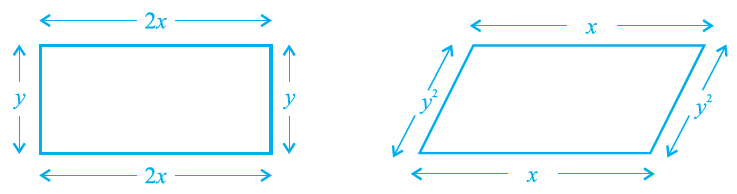We know that, perimeter of rectangle = 2 (length + breadth)

= 2 (2x + y)

= 4x + 2y

The unlike terms in perimeters of following figures are 2y and 2y2.

Perimeter of parallelogram = x + x + y2 + y2

= 2x + 2y2

30. On adding a monomial _____________ to – 2x + 4y2 + z, the resulting expression becomes a binomial.

Solution:-

On adding a monomial 2x or -4y2 or -z to – 2x + 4y2 + z, the resulting expression becomes a binomial.

2x + (-2x + 4y2 + z) = 2x – 2x + 4y2 + z

= 4y2 + z

-4y2 + (-2x + 4y2 + z) = -4y2 – 2x + 4y2 + z

= – 2x + z

-z + (-2x + 4y2 + z) = -z – 2x + 4y2 + z

= -2x + 4y2

31. 3x + 23x2 + 6y2 + 2x + y2 + ____________ = 5x + 7y2.

Solution:-

3x + 23x2 + 6y2 + 2x + y2 + (-23x2) = 5x + 7y2.

Let us consider the missing letter be p.

Then,

3x + 23x2 + 6y2 + 2x + y2 + p = 5x + 7y2

By transposing 3x, 23x2, 6y2, 2x and y2 to RHS

5x – 3x – 23x2 + 7y2 – 6y2 – 2x – y2 = p

2x – 2x – 23x2 + y2 – y2 = p

p = 0 – 23x2 – 0

p = – 23x2

32. If Rohit has 5xy toffees and Shantanu has 20yx toffees, then Shantanu has _____ more toffees.

Solution:-

If Rohit has 5xy toffees and Shantanu has 20yx toffees, then Shantanu has 15xy more toffees.

From the question,

Rohit has 5xy toffees

Shantanu has 20yx toffees

Then, difference between the toffees of both Rohit and Shantanu = 20yx – 5xy = 15 xy

Then Shantanu has 15 xy more toffees.

In questions 33 to 52, state whether the statements given are True or False.

33. 1 + (x/2) + x3 is a polynomial

Solution:-

True.

In general, an expression with one or more than one term (with nonnegative integral exponents of the variables) is called a ‘Polynomial’.

34. (3a – b + 3) – (a + b) is a binomial.

Solution:-

False.

Consider the given expression,

(3a – b + 3) – (a + b)

3a – b + 3 – a – b

2a – 2b + 3

Therefore, the given expression contains 3 terms.

So, it is a trinomial.

35. A trinomial can be a polynomial.

Solution:-

True.

In general, an expression with one or more than one term (with nonnegative integral exponents of the variables) is called a ‘Polynomial’.

36. A polynomial with more than two terms is a trinomial.

Solution:-

False.

Expression with three unlike terms is called a ‘Trinomial’.

37. Sum of x and y is x + y.

Solution:-

True.

38. Sum of 2 and p is 2p.

Solution:-

False.

Sum of 2 and p is 2 + p.

39. A binomial has more than two terms.

Solution:-

False

Expression with two unlike terms is called a ‘Binomial’

40. A trinomial has exactly three terms.

Solution:-

True.

Expression with three unlike terms is called a ‘Trinomial’.

41. In like terms, variables and their powers are the same.

Solution:-

True.

The terms having the same algebraic factors are called like terms.

42. The expression x + y + 5x is a trinomial.

Solution:-

False.

Consider the given expression, x + y + 5x

The expression contains like terms, x + 5x = 6x

Then, the given expression becomes y + 6x which is a binomial.

43. 4p is the numerical coefficient of q2 in – 4pq2.

Solution:-

False.

-4 is the numerical coefficient of q2 in – 4pq2.

44. 5a and 5b are unlike terms.

Solution:-

True.

The terms having different algebraic factors are called unlike terms.

45. Sum of x2 + x and y + y2 is 2x2 + 2y2.

Solution:-

False.

Sum of x2 + x and y + y2

= (x2 + x) + (y + y2)

= x2 + y2 + x + y

46. Subtracting a term from a given expression is the same as adding its additive inverse to the given expression.

Solution:-

True.

Additive inverse of the expression is same as the subtracting term.

47. The total number of planets of Sun can be denoted by the variable n.

Solution:-

False.

We know that, total number of planets is constant. Hence, we cannot denote planets in variables.

48. In like terms, the numerical coefficients should also be the same.

Solution:-

False.

The terms having the same algebraic factors are called like terms. Numerical of the coefficient can be vary.

49. If we add a monomial and binomial, then answer can never be a monomial.

Solution:-

False.

If we add a monomial and binomial, then answer can be a monomial.

For example: sum of y2 and –y2 + x2

= y2 + (-y2 + x2)

= y2 – y2 + x2

= x2

50. If we subtract a monomial from a binomial, then answer is at least a binomial.

Solution:-

False.

If we subtract a monomial from a binomial, then answer is at least a monomial.

For example: difference of y2 and y2 + x2

= y2 – (y2 + x2)

= y2 – y2 – x2

= – x2

51. When we subtract a monomial from a trinomial, then answer can be a polynomial.

Solution:-

True.

When we subtract a monomial from a trinomial, then answer can be binomial or polynomial.

For example: subtract p2 from p2 + q2 – r2

= (p2 + q2 – r2) – p2

= p2 + q2 – r2 – p2

= q2 – r2

52. When we add a monomial and a trinomial, then answer can be a monomial.

Solution:-

False.

When we add a monomial and a trinomial, then answer can be binomial or trinomial.

For example: add p2 and p2 + q2 – r2

= p2 + (p2 + q2 – r2)

= p2 + p2 + q2 – r2

= 2p2 + q2 – r2

53. Write the following statements in the form of algebraic expressions and write whether it is monomial, binomial or trinomial.

(a) x is multiplied by itself and then added to the product of x and y.

Solution:-

From the question it is given that,

x is multiplied by itself = x × x = x2

the product of x and y = x × y = xy

Then, As per the condition in the question = x2 + xy

Therefore, the obtained expression is binomial.

(b) Three times of p and two times of q are multiplied and then subtracted from r.

Solution:-

From the question it is given that,

Three times of p = 3p

Two times of q = 2q

Three times of p and two times of q are multiplied = 3p × 2q =3p2q

Then, As per the condition in the question = r – 3p2q

Therefore, the obtained expression is binomial.

(c) Product of p, twice of q and thrice of r.

Solution:-

As per the condition given in the question,

p × 2q × 3r = 6pqr

Therefore, the obtained expression is a monomial.

(d) Sum of the products of a and b, b and c and c and a.

Solution:-

The products of a and b, b and c and c and a = (a × b) and (b × c) and (c × a)

Then, sum of the products of a and b, b and c and c and a = ab + bc + ca

Therefore, the obtained expression is trinomial.

(e) Perimeter of an equilateral triangle of side x.

Solution:-

We know that, perimeter of triangle = sum of all sides

= x + x + x

= 3x

Therefore, the obtained expression is monomial.

(f) Perimeter of a rectangle with length p and breadth q.

Solution:-

We know that, perimeter of rectangle = 2 (length + breadth)

= 2 (p + q)

= 2p + 2q

Therefore, the obtained expression is binomial.

(g) Area of a triangle with base m and height n.

Solution:-

We know that, area of triangle = ½ × base × height

= ½ × m × n

= ½mn

Therefore, the obtained expression is monomial.

(h) Area of a square with side x.

Solution:-

We know that, area of square = side × side

= x × x

= x2

Therefore, the obtained expression is monomial.

(i) Cube of s subtracted from cube of t.

Solution:-

As per the condition given in the question, t3 – s3

Therefore, the obtained expression is binomial.

(j) Quotient of x and 15 multiplied by x.

Solution:-

Quotient of x and 15 = x ÷ 15

As per the condition given in the question, Quotient of x and 15 multiplied by

x = (x ÷ 15)x

= x2/15

Therefore, the obtained expression is monomial.

(k) The sum of square of x and cube of z.

Solution:-

As per the condition given in the question = x2 + z3

Therefore, the obtained expression is binomial.

(l) Two times q subtracted from cube of q.

Solution:-

As per the condition given in the question = q3 – 2q

Therefore, the obtained expression is binomial.

54. Write the coefficient of x2 in the following:

(i) x2 – x + 4

Solution:-

The coefficient of x2 in the given expression is 1.

Coefficient is the numerical factor in a term. Sometimes, any factor in a term is called the coefficient of the remaining part of the term.

(ii) x3 – 2x2 + 3x + 1

Solution:-

The coefficient of x2 in the given expression is -2.

Coefficient is the numerical factor in a term. Sometimes, any factor in a term is called the coefficient of the remaining part of the term.

(iii) 1 + 2x + 3x2 + 4x3

Solution:-

The coefficient of x2 in the given expression is 3.

Coefficient is the numerical factor in a term. Sometimes, any factor in a term is called the coefficient of the remaining part of the term.

(iv) y + y2x + y3x2 + y4x3

Solution:-

The coefficient of x2 in the given expression is y3.

Coefficient is the numerical factor in a term. Sometimes, any factor in a term is called the coefficient of the remaining part of the term.

55. Find the numerical coefficient of each of the terms :

(i) x3y2z, xy2z3, –3xy2z3, 5x3y2z, –7x2y2z2

Solution:-

Numerical coefficient of,

x3y2z = 1

xy2z3 = 1

–3xy2z3 = -3

5x3y2z = 5

–7x2y2z2 = -7

(ii) 10xyz, –7xy2z, –9xyz, 2xy2z, 2x2y2z

Solution:-

Numerical coefficient of,

10xyz = 10

–7xy2z = -7

–9xyz = -9

2xy2z = 2

2x2y2z = 2